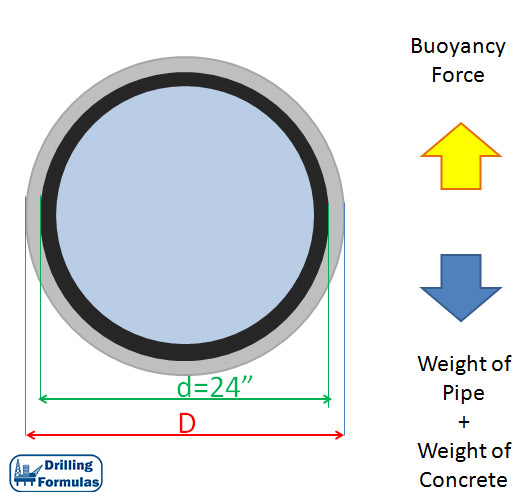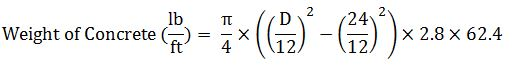# Determine Thickness of Concrete for Concrete Coated Pipeline

Coating the subsea pipeline with concrete is a technique to add downward force for stability of pipelines situated on the seabed. Figure 1 shows that the concrete coated subsea pipe line is being welded. This article will demonstrate how to calculate the thickness of concrete required in order to achieve the required net down force.Figure 1 – The Concrete Coated Subsea Pipe Line is being Welded

Determine thickness of coated cement based on the given information below.

Given Information

Pipeline size OD = 24” Inch

Weight of steel pipe/ft = 145 lb/ft

Fresh water density = 64.2 lb/cu-ft

Sea water density = 65.52 lb/cu-ft

Concrete SG = 2.8

Required net down force for pipeline stability = 200 lb/ftFigure 2 – Cross Section of Concrete Coated Pipeline

Force Diagram

Upwards force is buoyancy from the sea water.

Downwards force is weight of cement and pipeline.

Figure 3 shows the diagram of force acting on the pipe.Figure 3 – Force acting on the pipeline

Based on the requirement, the relationship can be written in the mathematical equation shown below;

200 = Weight of pipe + Weight of Concrete – Buoyancy Force

Weight of pipe = 145 lb/ft

Weight of Concrete = Volume of concrete per ft × Density of concrete

Weight of Concrete = Volume of concrete per ft × SG of concrete × Water DensityBuoyancy Force = Volume of coated pipeline per ft × Density of sea waterSolve for D;

D = 31.8 inch

Total diameter is 31.8 inch.

Thickness of cement required = (31.8-24) ÷ 2 = 3.9 inch

The method to coat the pipe line is shown as per the video below.

Share the joy
Tagged , , , , . Bookmark the permalink.